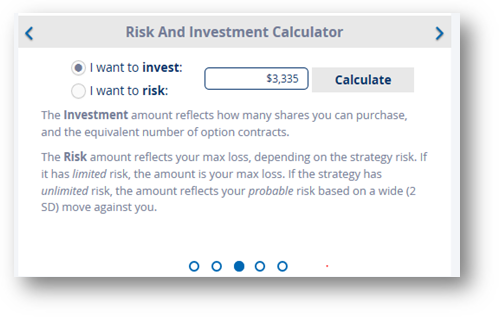Risk and Investment Calculator

• Investment Calculator : Calculates the # of shares you can buy based on the investment amount and defaults to an equivalent number of contracts in an options strategy. This provides a good comparison of options strategies to an underlying share purchases/sales. For example, if you have enough money to purchase 300 shares of XYZ, the options strategy will have a quantity of 3 contracts to provide a good comparison.
• Risk Calculator : Calculates the # of contracts for each strategy where the Max Risk does not exceed the amount the user enters. If the strategy has unlimited risk, the max risk at 2 Standard Deviations is used for this calculation. (Same as VaR calculations with 95% confidence)